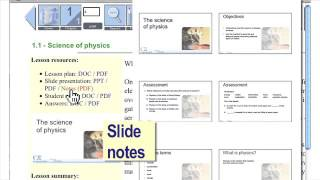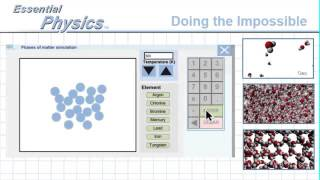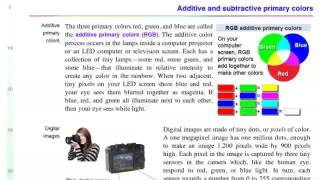# Translating between Math and Physics - Essential Physics (1st Edition)

Help students understand algebra and apply it to the real world.

Mathematics is the language of science but the DIALECT of math spoken in math class is different from science class. The equation of a line is y = mx + b, right? Well, v = v0 + at is ALSO the equation for a straight line in the dialect of physics. Essential Physics provides clear graphical translations from math class math to real math applications in the text as well as in both the slides and student assignments.

### More "PASCO Essential Science" Videos